# CBSE Class 10th Maths Question Paper Solved 2017 18 19 20 With Solutions

Here we are providing CBSE Previous Year Question Papers Class 6 to 12 solved with soutions CBSE Class 10th Maths Question Paper Solved 2017 18 19 20 with solutions class 10 Maths sample paper 2020 solved, sample paper class 10 Maths, cbse class 10 Maths question paper 2018, sample paper class 10 Maths 2019, Maths question paper for class 10, cbse previous year question papers class 10 Maths, cbse sample paper f Practice of previous year question papers and sample papers protects each and every student to score bad marks in exams.If any student of CBSE Board continuously practices last year question paper student will easily score high marks in tests. Fortunately earlier year question papers can assist the understudies with scoring great in the tests. Unraveling previous year question paper class 10 Maths is significant for understudies who will show up for Class 10 Board tests.

## Class 10 Subject Maths Paper Set 4 with Solutions

SECTION A

Question numbers 1 to 20 carry 1 mark each.

Choose the correct option in question numbers 1 to 10

Question 1: (Marks 1)

Given that HCF (156, 78) = 78, LCM (156, 78) is

(A) 156

(B) 78

(C) 156 × 78

(D) 156 × 2

Answer :

(A) 156

Question 2: (Marks 1)

Sides of two similar triangles are in the ratio 4 : 9. Areas of these triangles are in the ratio

(A) 4 : 9

(B) 2 : 3

(C) 81 : 16

(D) 16 : 81

Answer :

(D) 16 : 81

Question 3: (Marks 1)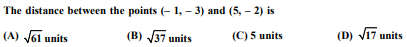Answer :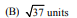Question 4: (Marks 1)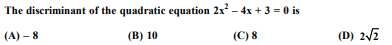OR

Roots of the quadratic equation 2x2 – 4x + 3 = 0 are

(A) real and equal

(B) real and distinct

(C) not real

(D) real

Answer :

(A) –8

OR

(C) Not Real

Question 5: (Marks 1)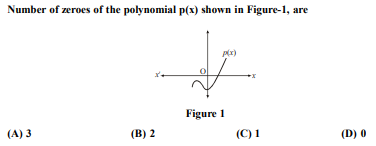Answer :

(C) 1

Question 6: (Marks 1)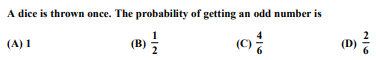Answer :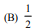Question 7: (Marks 1)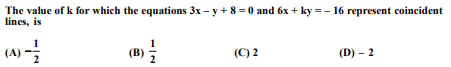Answer :

(D) –2

Question 8: (Marks 1)

If sin A = cos A, 0 < A < 90°, then the angle A is equal to

(A) 30°

(B) 60°

(C) 0°

(D) 45°

Answer :

(D) 45°

Question 9: (Marks 1)

The second term from the end of the A.P. 5, 8, 11, ..., 47 is

(A) 50

(B) 45

(C) 44

(D) 41

Answer :

(C) 44

Question 10: (Marks 1)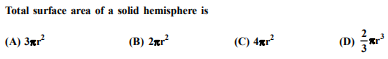Answer :

(A) 3πr2

Fill in the blanks in question numbers 11 to 15.

Question 11: (Marks 1)

The roots of the equation, x2 + bx + c = 0 are equal if _______.

Answer :

b2 = 4c

Question 12: (Marks 1)

The mid-point of the line segment joining the points (– 3, – 3) and (– 3, 3) is _______.

Answer :

(–3, 0)

Question 13: (Marks 1)

The lengths of the tangents drawn from an external point to a circle are _______.

Answer :

Equal

Question 14: (Marks 1)

For a given distribution with 100 observations, the ’less than’ ogive and ’more than’ ogive intersect at (58, 50). The median of the distribution is _______.

Answer :

58

Question 15: (Marks 1)

In the quadratic polynomial t2 – 16, sum of the zeroes is _______.

Answer :

0

Answer the following question numbers 16 to 20.

Question 16: (marks 1)

Write the 26th term of the A.P. 7,4,1, –2, ... .

Answer :

d = –3

a26 = –68

Question 17: (Marks 1)

Find the coordinates of the point on x-axis which divides the line segment joining the points (2, 3) and (5, – 6) in the ratio 1 : 2.

Answer :

Let the point on x-axis be (x, 0)

∴ Required point is (3, 0)

Question 18: (Marks 1)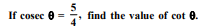OR

Find the value of sin 42° – cos 48°.

Answer :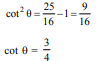OR

sin 42° = cos (90° – 42°) = cos 48°

∴ sin 42° – cos 48° = 0

Question 19: (Marks 1)

The angle of elevation of the top of the tower AB from a point C on the ground, which is 60 m away from the foot of the tower, is 30°, as shown in Figure-2. Find the height of the tower.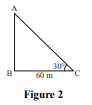Answer :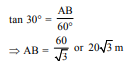Question 20: (Marks 1)

In Figure-3, find the length of the tangent PQ drawn from the point P to a circle with centre at O, given that OP = 12 cm and OQ = 5 cm. P Q O 5 cm 12 cm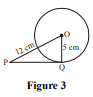Answer :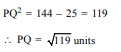SECTION B

Question numbers 21 to 26 carry 2 marks each.

Question 21: (Marks 2)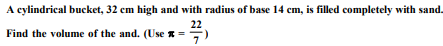Answer :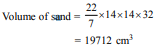Question 22: (Marks 2)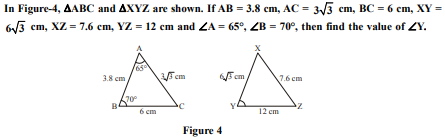OR

If the areas of two similar triangles are equal, show that they are congruent.

Answer :

ΔABC ~ ΔXZY

⇒ ∠Y = ∠C = 180° – (∠A + ∠B)

⇒ ∠Y = 45º

OR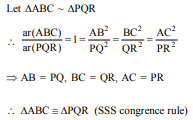Question 23: (Marks 2)

If sec 2A = cosec (A – 30°), 0° < 2A < 90°, then find the value of ∠A.

Answer :

sec 2A = cosec (A – 30°)

⇒ cosec (90° – 2A) = A – 30°

⇒ 90º – 2A = A – 30°

⇒ ∠A = 40º

Question 24: (Marks 2)

Show that every positive even integer is of the form 2q and that every positive odd integer is of the form 2q + 1, where q is some integer.

Answer :

Let ‘a’ be any positive integer and b = 2

Using Euclid’s Division lemma

a = 2q + r, r = 0,

∴ a = 2q or 2q + 1

Because 2q is an even integer and every positive ineger is either even or odd.

∴ a = 2q + 1 is an odd positive integer.

Question 25: (Marks 2)

How many two-digit numbers are divisible by 6?

OR

In an A.P. it is given that common difference is 5 and sum of its first ten terms is 75. Find the first term of the A.P.

Answer :

Two digit numbers divisible by 6 are 12, 18, 24,...96

96 = 12 + (n – 1) × 6

⇒ n = 15

OR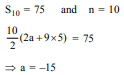Question 26: (Marks 2)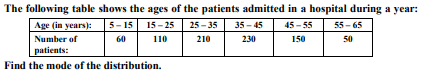Answer :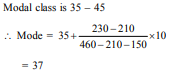SECTION C

Question numbers 27 to 34 carry 3 marks each.

Question 27: (Marks 3)

Seema has a 10 m × 10 m kitchen garden attached to her kitchen. She divides it into a 10 × 10 grid and wants to grow some vegetables and herbs used in the kitchen. She puts some soil and manure in that and sows a green chilly plant at A, a coriander plant at B and a tomato plant at C.
Her friend Kusum visited the garden and praised the plants grown there. She pointed out that they seem to be in a straight line. See the below diagram carefully and answer the following questions: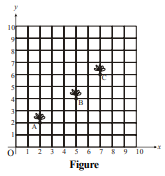(i) Write the coordinates of the points A, B and C taking the 10 × 10 grid as coordinate axes.

(ii) By distance formula or some other formula, check whether the points are collinear.

Answer :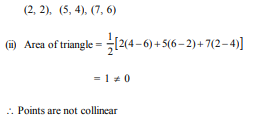Question 28: (Marks 3)

In Figure-5, a circle is inscribed in a ΔABC touching BC, CA and AB at P, Q and R respectively. If AB = 10 cm, AQ = 7 cm, CQ = 5 cm, find the length of BC.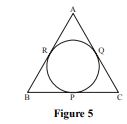OR

In Figure-6, two tangents TP and TQ are drawn to a circle with centre O from an external point T. Prove that ∠PTQ = 2 ∠OPQ.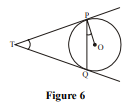Answer :

AR = AQ = 7 cm

BR = AB – AR = 10 – 7 = 3 cm

BC = BP + PC

= BR + CQ

= 3 + 5 = 8 cm

OR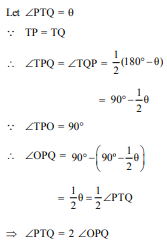Question 29: (Marks 3)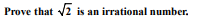Answer :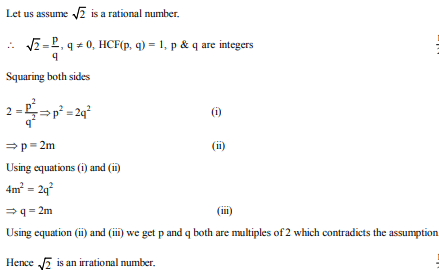Question 30: (Marks 3)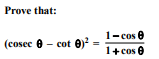Answer :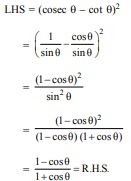Question 31: (Marks 3)

5 pencils and 7 pens together cost ` 250 whereas 7 pencils and 5 pens together cost ` 302. Find the cost of one pencil and that of a pen.

OR

Solve the following pair of equations using cross-multiplication method:

x – 3y – 7 = 0

3x – 5y – 15 = 0

Answer :

Let the cost of 1 pencil be ` x and cost of 1 pen be ` y.

5x + 7y = 250 ...(i)

7x + 5y = 302 ...(ii)

Solving (i) and (ii)

x = 36 and y = 10

Hence, cost of 1 pencil = ` 36

cost of 1 pen = ` 10

OR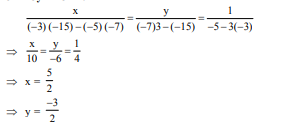Question 32: (Marks 3)

One card is drawn from a well-shuffled deck of 52 cards. Find the probability of getting

(i) a king of red colour.

(ii) the queen of diamonds.

(iii) an ace.

OR

A box contains 90 discs which are numbered from 1 to 90. If one disc is drawn at random from the box, find the probability that it bears

(i) a two-digit number.

(ii) a perfect square number.

(iii) a prime, number less than 15.

Answer :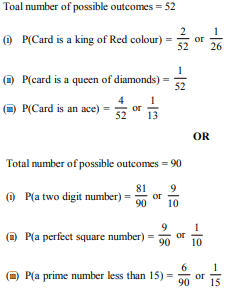Question 33: (Marks 3)

In Figure-7, ABCD is a square of side 14 cm. From each corner of the square, a quadrant of a circle of radius 3.5 cm is cut and also a circle of radius 4 cm is cut as shown in the figure. Find the area of the remaining (shaded) portion of the square.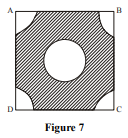Answer :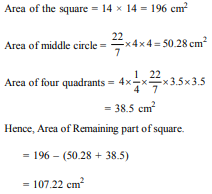Question 34: (Marks 3)

Draw a circle of radius 3 cm. Take a point P outside the circle at a distance of 7 cm from the centre O of the circle and draw two tangents to the circle.

Answer :

Drawing a circle and locating point P.

Constructing tangents from P.

Question 35: (Marks 4)

In a right-angled triangle, prove that the square of the hypotenuse is equal to the sum of the squares of the remaining two sides.

Answer :

For correct given, to prove, figure and construction.

Correct proof

Question 36: (Marks 4)

Divide polynomial – x3 + 3x2 – 3x – 3x + 5 by the polynomial x2 + x – 1 and verify the division algorithm.

OR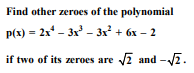Answer :

On dividing –x3 + 3x2 – 3x + 5 by x2 + x – 1

We get quotient = –x + 4

and Remainder = –8x + 9

Verification

(x2 + x – 1) (–x + 4) + (9 – 8x)

= x3 - x2 + x + 4x2 + 4x - 4 + 9 - 8x

= -x3 + 3x2 - 3x + 5

OR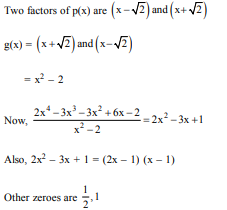Question 37: (Marks 4)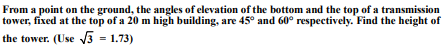Answer :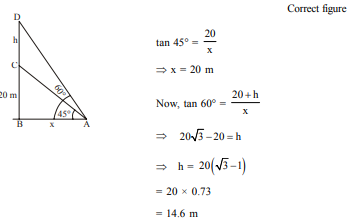Question 38: (Marks 4)

A bucket is in the form of a frustum of a cone of height 30 cm with the radii of its lower and upper circular ends as 10 cm and 20 cm respectively. Find the capacity of the bucket. (Use π = 3.14)

OR

Water in a canal 6 m wide and 1.5 m deep, is flowing with a speed of 10 km/hr. How much area will it irrigate in 30 minutes if 4 cm of standing water is needed?

Answer :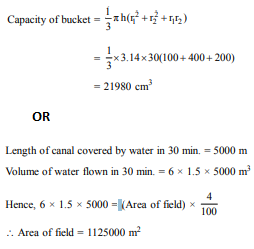Question 39: (Marks 4)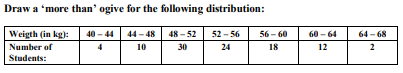Answer :

Points to be plotted for more than type ogive

(40, 100), (44, 96), (48, 86), (52, 56), (56, 32), (60, 14), (64, 2)

For drawing correct ogive

Question 40: (Marks 4)

A train travels 360 km at a uniform speed. If the speed had been 5 km/hr more, it would have taken 1 hour less for the same journey. Find the original speed of the train.

OR

Sum of the areas of two squares is 468 m2 . If the difference of their parameters is 24 m, find the sides of the two squares.

Answer :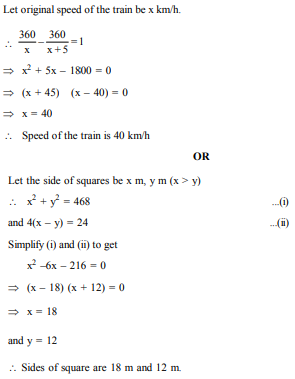☞ Click here for privious year Question papers

## class 10 Maths sample paper 2020 solved, sample paper class 10 Maths, cbse class 10 Maths question paper 2018, sample paper class 10 Maths 2019, Maths question paper for class 10, cbse previous year question papers class 10 Maths, cbse sample paper for class 10 Maths, cbse class 10 Maths question paper 2019, class 10 Maths sample paper 2019 solved, cbse class 10th Maths question paper, 10th Maths question paper, sample paper class 10 Maths 2020, Maths sample paper class 12, cbse question paper for class 9 Maths, cbse Maths sample paper, cbse 10th class Maths

### NCERT Books Free Pdf Download for Class 5, 6, 7, 8, 9, 10 , 11, 12 Hindi and English Medium

 Mathematics Biology Psychology Chemistry English Economics Sociology Hindi Business Studies Geography Science Political Science Statistics Physics Accountancy

## Please Share this webpage on facebook, whatsapp, linkdin and twitter.

Copyright @ ncerthelp.com A free educational website for CBSE, ICSE and UP board.